• 累计撰写 37 篇文章
• 累计收到 35 条评论

# 使用JSDelivr的全球免费CDN免费加速静态资源2021-01-26 / 1 评论 / 147 阅读 / 正在检测是否收录...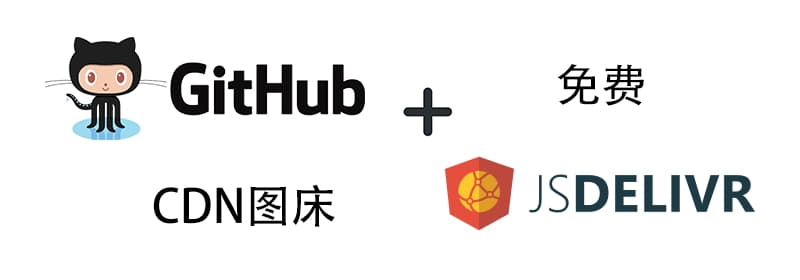# 一、介绍

## 1. CDN

CDN的全称是`Content Delivery Network`，即内容分发网络。CDN是构建在网络之上的内容分发网络，依靠部署在各地的边缘服务器，通过中心平台的负载均衡、内容分发、调度等功能模块，使用户就近获取所需内容，降低网络拥塞，提高用户访问响应速度和命中率。CDN的关键技术主要有内容存储和分发技术。

## 2. jsDelivr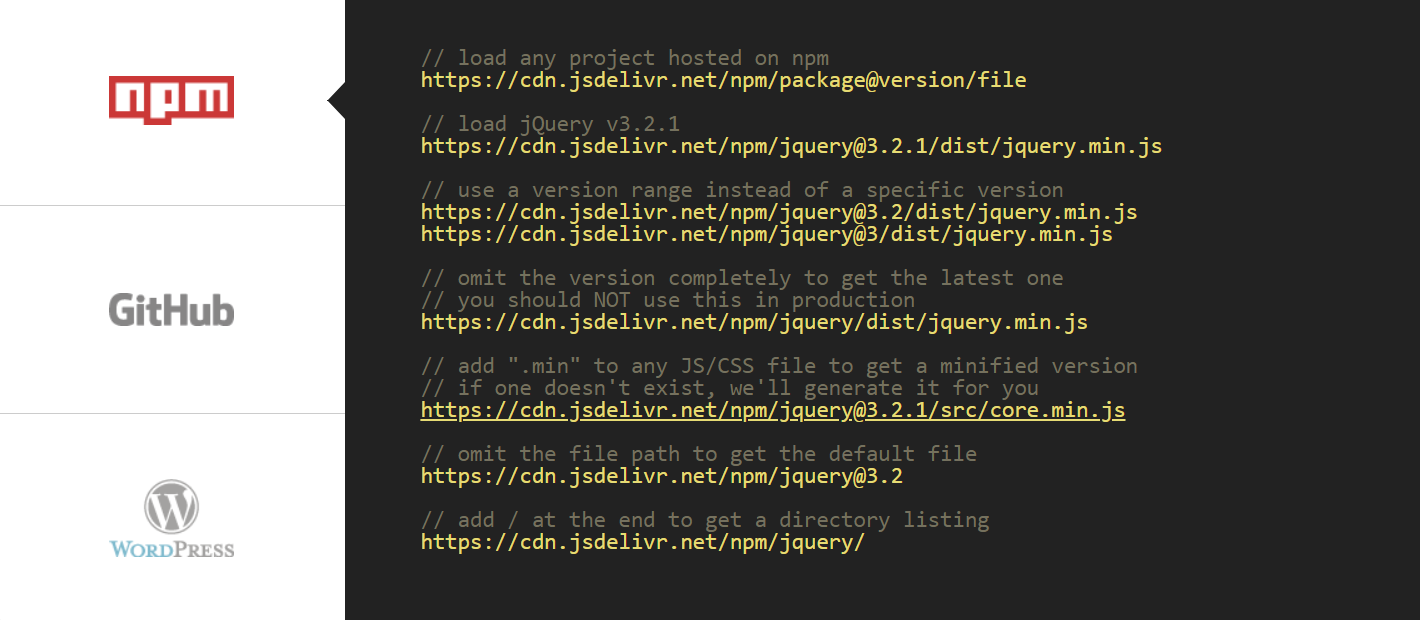jsDelivr 是一个提供含JavaScript库、jQuery插件、CSS框架、字体等Web上常用静态资源的免费开源的 CDN 解决方案。其采用全球CDN加速，确保每个地区的使用者都能获得最好的连接速度，而在大陆地区使用了国内的CDN加速。因此jsDelivr在大陆也有很好的相应速度，jsDelivr可将不同的JavaScript或CSS libraries集合在一起使用，同时，jsDelivr也提供包括`npm``GitHub``WordPress`等项目的镜像服务。

## 3. GitHub

gitHub是一个面向开源及私有软件项目的托管平台，因为只支持git 作为唯一的版本库格式进行托管，故名GayHub GitHub。其使用方法网上已有许多教程，在此不再赘述。

# 二、使用

## 1. 新建GitHub仓库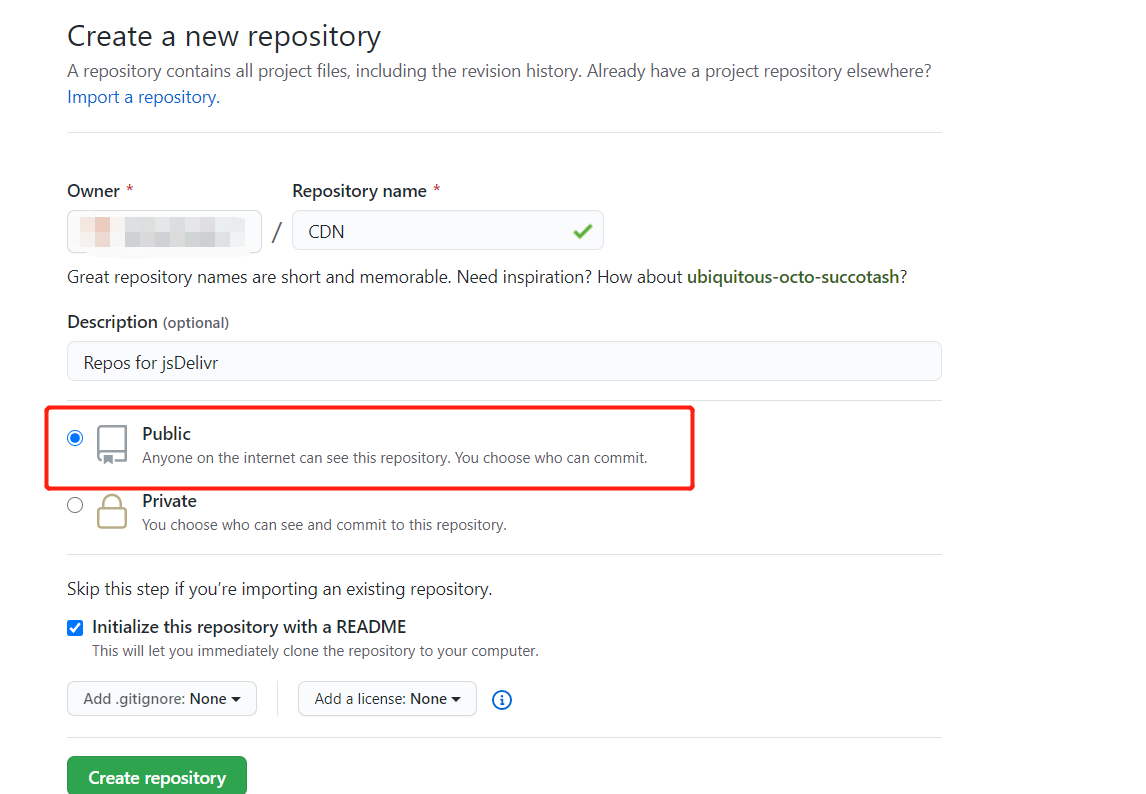## 2. 克隆仓库到本地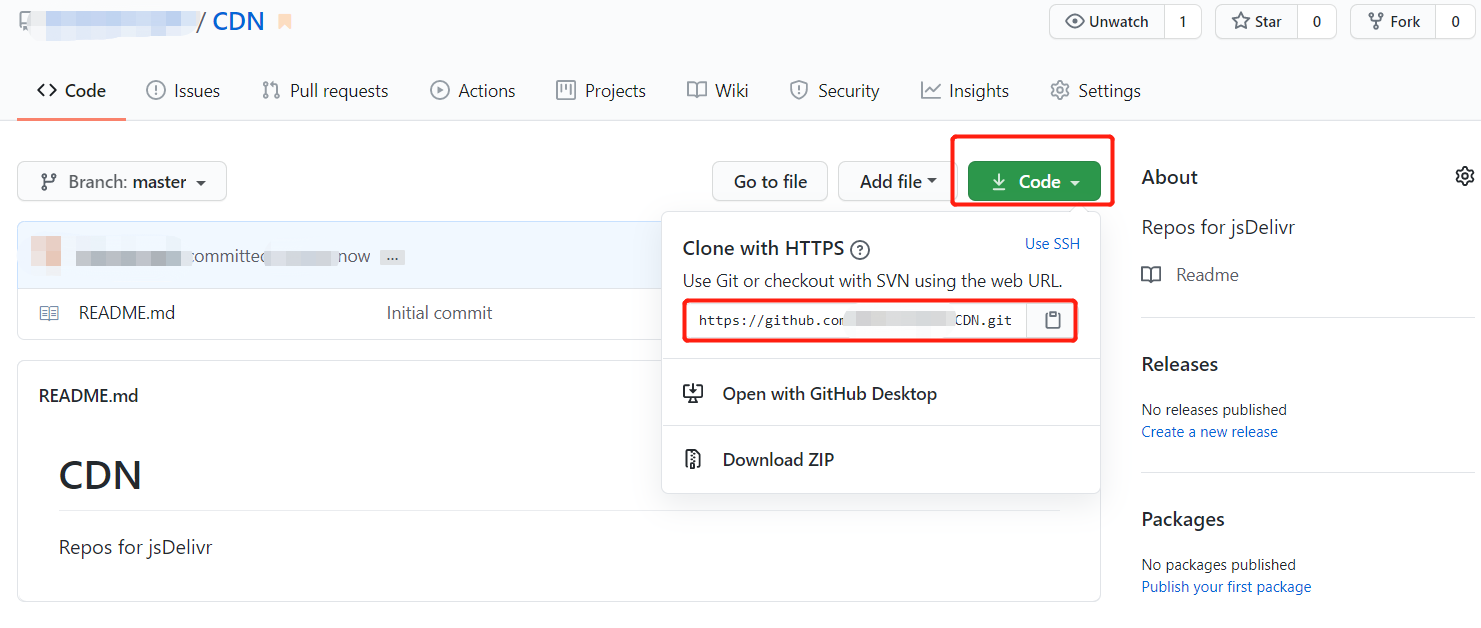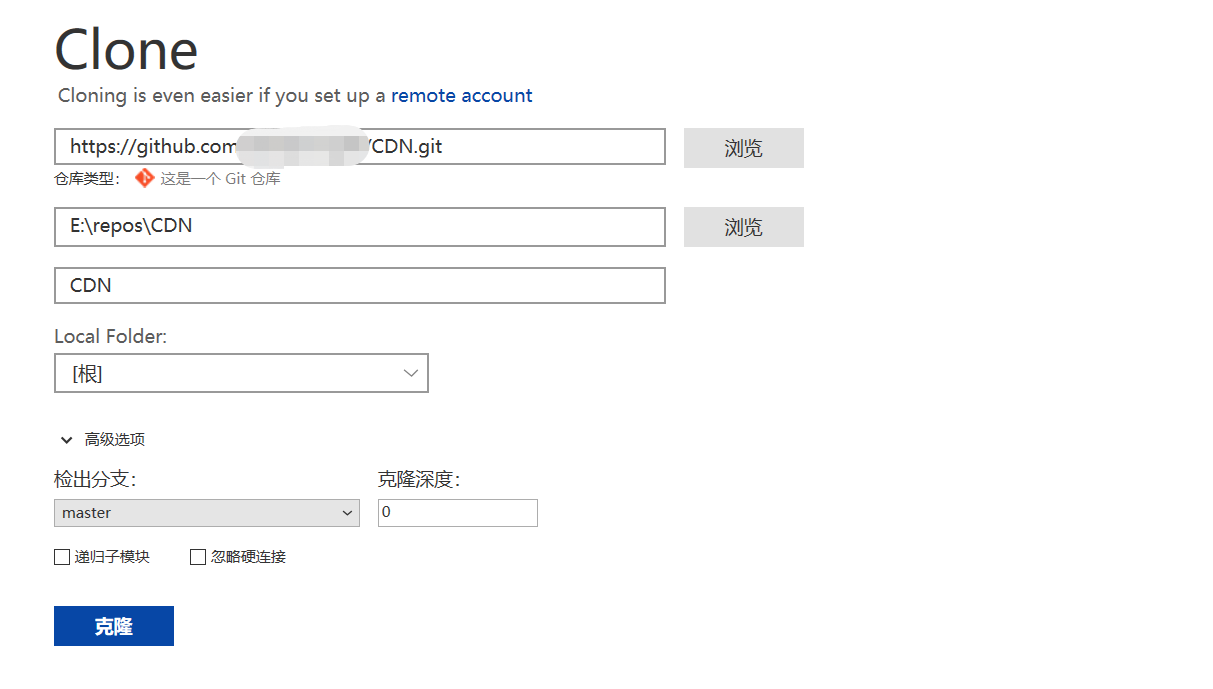## 3. 提交静态资源到GitHub

PS：jsDelivr不支持加载超过20M的资源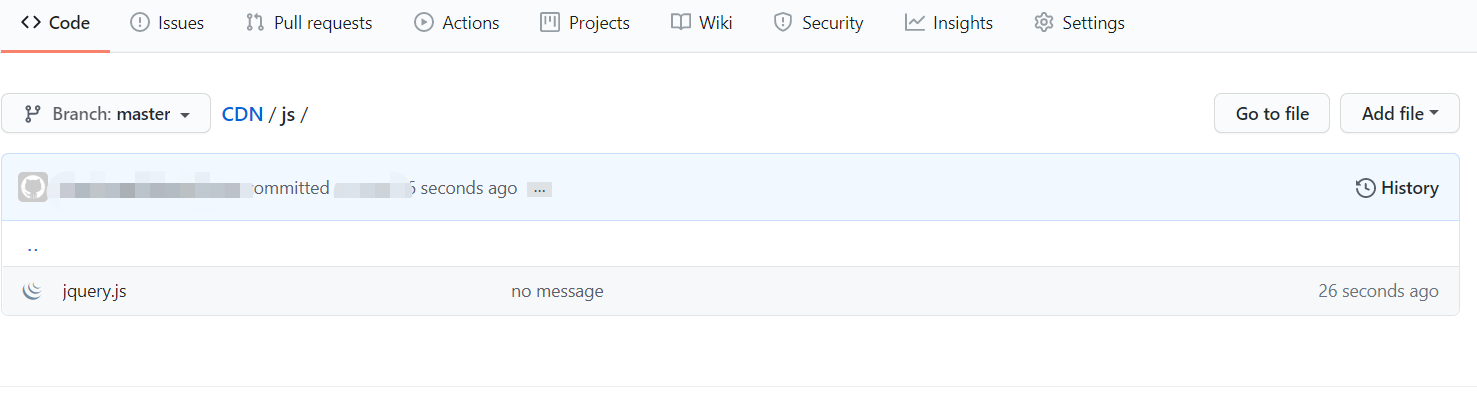## 5. 其它使用方法

jsdelivr的版本号不是必需的，是为了区分新旧资源，如果不使用版本号，将会直接引用最新资源，除此之外还可以使用某个范围内的版本，查看所有资源等，具体使用方法如下：

``````
// 根据版本号、commit、branch引用
https:[email protected]/* <![CDATA[ */!function(t,e,r,n,c,a,p){try{t=document.currentScript||function(){for(t=document.getElementsByTagName('script'),e=t.length;e--;)if(t[e].getAttribute('data-yjshash'))return t[e]}();if(t&&(c=t.previousSibling)){p=t.parentNode;if(a=c.getAttribute('data-yjsemail')){for(e='',r='0x'+a.substr(0,2)|0,n=2;a.length-n;n+=2)e+='%'+('0'+('0x'+a.substr(n,2)^r).toString(16)).slice(-2);p.replaceChild(document.createTextNode(decodeURIComponent(e)),c)}p.removeChild(t)}}catch(u){}}()/* ]]> *//file

// 例子：
// 版本号
https:[email protected]/* <![CDATA[ */!function(t,e,r,n,c,a,p){try{t=document.currentScript||function(){for(t=document.getElementsByTagName('script'),e=t.length;e--;)if(t[e].getAttribute('data-yjshash'))return t[e]}();if(t&&(c=t.previousSibling)){p=t.parentNode;if(a=c.getAttribute('data-yjsemail')){for(e='',r='0x'+a.substr(0,2)|0,n=2;a.length-n;n+=2)e+='%'+('0'+('0x'+a.substr(n,2)^r).toString(16)).slice(-2);p.replaceChild(document.createTextNode(decodeURIComponent(e)),c)}p.removeChild(t)}}catch(u){}}()/* ]]> *//dist/jquery.js

// 版本区间
https:[email protected]/* <![CDATA[ */!function(t,e,r,n,c,a,p){try{t=document.currentScript||function(){for(t=document.getElementsByTagName('script'),e=t.length;e--;)if(t[e].getAttribute('data-yjshash'))return t[e]}();if(t&&(c=t.previousSibling)){p=t.parentNode;if(a=c.getAttribute('data-yjsemail')){for(e='',r='0x'+a.substr(0,2)|0,n=2;a.length-n;n+=2)e+='%'+('0'+('0x'+a.substr(n,2)^r).toString(16)).slice(-2);p.replaceChild(document.createTextNode(decodeURIComponent(e)),c)}p.removeChild(t)}}catch(u){}}()/* ]]> *//dist/jquery.js
https:[email protected]/* <![CDATA[ */!function(t,e,r,n,c,a,p){try{t=document.currentScript||function(){for(t=document.getElementsByTagName('script'),e=t.length;e--;)if(t[e].getAttribute('data-yjshash'))return t[e]}();if(t&&(c=t.previousSibling)){p=t.parentNode;if(a=c.getAttribute('data-yjsemail')){for(e='',r='0x'+a.substr(0,2)|0,n=2;a.length-n;n+=2)e+='%'+('0'+('0x'+a.substr(n,2)^r).toString(16)).slice(-2);p.replaceChild(document.createTextNode(decodeURIComponent(e)),c)}p.removeChild(t)}}catch(u){}}()/* ]]> *//dist/jquery.js

// 完全省略该版本以获取最新版本
https://cdn.jsdelivr.net/gh/jquery/jquery/dist/jquery.js

// 将“.min”添加到任何JS/CSS文件中以获取缩小版本，如果不存在，将为会自动生成
https:[email protected]/* <![CDATA[ */!function(t,e,r,n,c,a,p){try{t=document.currentScript||function(){for(t=document.getElementsByTagName('script'),e=t.length;e--;)if(t[e].getAttribute('data-yjshash'))return t[e]}();if(t&&(c=t.previousSibling)){p=t.parentNode;if(a=c.getAttribute('data-yjsemail')){for(e='',r='0x'+a.substr(0,2)|0,n=2;a.length-n;n+=2)e+='%'+('0'+('0x'+a.substr(n,2)^r).toString(16)).slice(-2);p.replaceChild(document.createTextNode(decodeURIComponent(e)),c)}p.removeChild(t)}}catch(u){}}()/* ]]> *//src/core.min.js

// JavaScript或CSS libraries合并使用
https:[email protected]/* <![CDATA[ */!function(t,e,r,n,c,a,p){try{t=document.currentScript||function(){for(t=document.getElementsByTagName('script'),e=t.length;e--;)if(t[e].getAttribute('data-yjshash'))return t[e]}();if(t&&(c=t.previousSibling)){p=t.parentNode;if(a=c.getAttribute('data-yjsemail')){for(e='',r='0x'+a.substr(0,2)|0,n=2;a.length-n;n+=2)e+='%'+('0'+('0x'+a.substr(n,2)^r).toString(16)).slice(-2);p.replaceChild(document.createTextNode(decodeURIComponent(e)),c)}p.removeChild(t)}}catch(u){}}()/* ]]> *//dist/jquery.min.js,[email protected]/* <![CDATA[ */!function(t,e,r,n,c,a,p){try{t=document.currentScript||function(){for(t=document.getElementsByTagName('script'),e=t.length;e--;)if(t[e].getAttribute('data-yjshash'))return t[e]}();if(t&&(c=t.previousSibling)){p=t.parentNode;if(a=c.getAttribute('data-yjsemail')){for(e='',r='0x'+a.substr(0,2)|0,n=2;a.length-n;n+=2)e+='%'+('0'+('0x'+a.substr(n,2)^r).toString(16)).slice(-2);p.replaceChild(document.createTextNode(decodeURIComponent(e)),c)}p.removeChild(t)}}catch(u){}}()/* ]]> *//dist/js/bootstrap.min.js
// 在末尾添加 / 以获取资源目录列表
https://cdn.jsdelivr.net/gh/jquery/jquery/``````

# 三、其它

jsDeliv官网：https://www.jsdelivr.com

5

### 评论 (1)

1.Windows 10 · Google Chrome回复